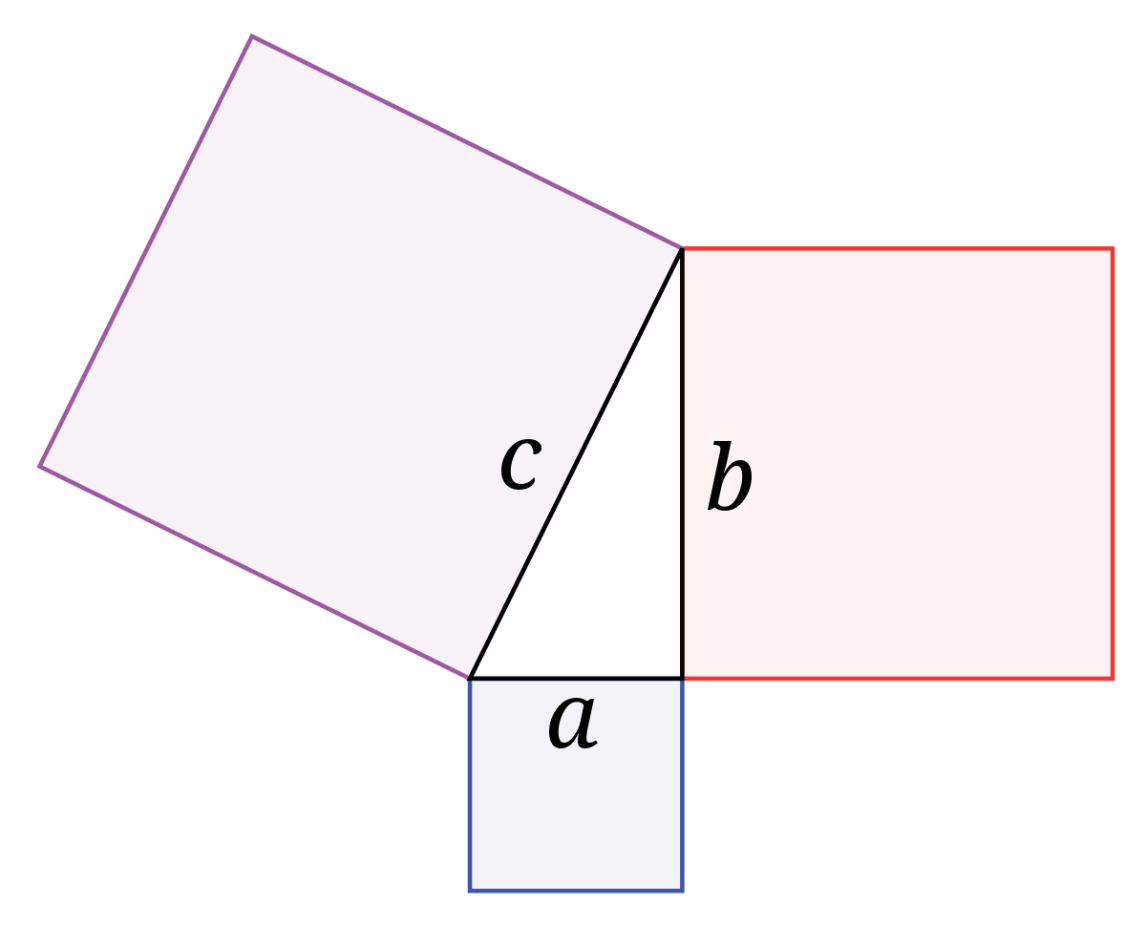# Right Triangles

Author:
Tosh

Describe any relationship you see between the measurements of the sides of the triangles, as well as the areas of the three squares?

Write an equation that relates a, b, c.

If a=3 and b=4, what would side c equal? Find the answer and re-create this in your figure.

Find the are of the largest square. Then find the length of just one side of the largest square.

Find the area of the two smaller squares in the diagram.

When you found the length of just one side of the three squares. How does this help me find the length of the side of a right triangle?

## Instructions

1. Open a new GeoGebra Classic window
2. Go to Options and select “Labeling”,check all new objects
3. Construct a Line Segment AB
4. Construct Perpendicular line through Point A, Line Segment AB
5. Construct Point C, anywhere on the perpendicular line
6. Hide the Perpendicular Line
7. Construct a Line Segment AC
8. Construct a square BC, by using the Regular Polygon Tool
9. Repeat Step 7 to create squares from Line Segment AC and Line Segment AB
10. Check Work: Is all your squares outside the triangle? If answer is No click undo and try selecting points opposite of the order.
11. Show Interior Angles of the Right Triangle.
12. Rename the sides “A, B, C” to match the diagram
13. Measure the length of A, B,C
14. Measure the area of each square
15. Show the Name and Value of “A, B, C”
16. Drag B around and observe the measurements and areas you have just created.
17. Set the font size of the text-box to Medium
18. Set text-box to Absolute Position on the screen
19. Set font size for the entire file to 18
20. Save the with the file name: LastName_GeoGebra.ggb; On the Web version, you need to go to the menu and download file to ggb file.

## Pythagorean Theorem